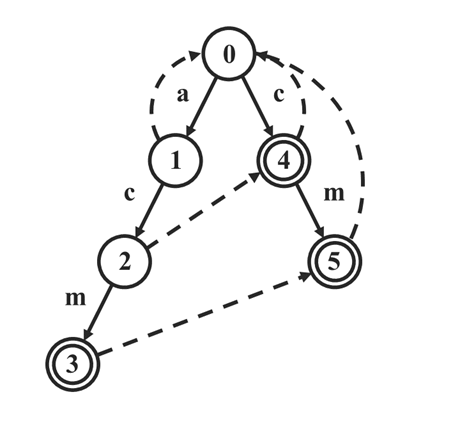# 【HDU2222 Keywords Search】AC自动机 | Trie | fail链 | E

## 【HDU 2222】Keywords Search

Time Limit: 2000/1000 MS (Java/Others)     Memory Limit: 131072/131072 K (Java/Others)
Total Submission(s): 81175    Accepted Submission(s): 28317

Tags：AC自动机 Trie树

### 输入

T T 组。第一行输入组数 T T

### 输入样例

1
5
she
he
say
shr
her
yasherhs



### 输出样例

3



### 分析

A C AC 自动机裸题。（然鹅也有坑点）

• 采用数组型 Trie树（类似链式前向星）而不是多次动态内存分配（同理在 B F S BFS 时自写 q u e u e queue ）。
• （参考于白书 P 216 P216 BFS 求失配指针时，不妨顺便把失配指针当作不存在的边给补上，以消除不必要的循环（可理解为记忆化

int match(const char *s)
{
int cnt = 0;
int now = ROOT;
for (const char *p=s; *p; ++p)
{
now = t[now].next[*p - 'a'];
cnt += t[now].cnt;
t[now].cnt;	// 避免模式串重复计数
}

return cnt;
}


（首先说明：问题不是出在没有考虑失配上，因为利用白书上的那个优化后失配指针已经成为了树上实实在在的边了，上面那样写和使用失配指针是等价的。）• ① 是当前串的后缀（根据 f a i l fail 指针定义）
• ② 是某个模式串的前缀（根据 T r i e Trie 树，即前缀树的定义）

###### 时间复杂度：
• Trie树 O ( N × L E N ( P ) ) O(N \times LEN(P)) 的。
• BFS，也是 O ( N × L E N ( P ) ) O(N \times LEN(P)) 的。
• 匹配文本串，喜闻乐见 O ( L E N ( T ) ) O(LEN(T)) 的。
• 遍历 f a i l fail 链，最坏最坏均摊 O ( N × L E N ( P ) ) O(N \times LEN(P)) 的。
• 总时间复杂度 O ( N × L E N ( P ) + L E N ( T ) ) O(N \times LEN(P) + LEN(T))

### AC代码

A C AC 一时爽， A C AC 自动机一直爽：

#include <cstdio>
#include <cstring>
#define sc(x) {register char _c=getchar(),_v=1;for(x=0;_c<48||_c>57;_c=getchar())if(_c==45)_v=-1;for(;_c>=48&&_c<=57;x=(x<<1)+(x<<3)+_c-48,_c=getchar());x*=_v;}

constexpr int ML(1e6+6);
constexpr int MN(5e5+5);
constexpr int MA(26);

class AC
{
private:

const int ROOT = 0;
struct
{
int next[MA], fail;
int cnt;
} t[MN];	// trie树
int tot;
int queue[MN];

public:

inline void init(void)
{
tot = 0;
memset(t, 0, sizeof(t));
t[ROOT].fail = -1;
}

void insert(const char *s)
{
int now = ROOT;
for (const char *p=s; *p; ++p)
{
if (!t[now].next[*p - 'a'])
t[now].next[*p - 'a'] = ++tot;
now = t[now].next[*p - 'a'];
}
++t[now].cnt;
}

void build(void)
{
int head = 0, tail = 0;
for (int i=0; i<MA; ++i)
if (t[ROOT].next[i])
queue[tail++] = t[ROOT].next[i], t[t[ROOT].next[i]].fail = 0;

{
for (int i=0; i<MA; ++i)
{
if (t[fa].next[i])
{
queue[tail++] = t[fa].next[i];
t[t[fa].next[i]].fail = t[t[fa].fail].next[i];
}
else
t[fa].next[i] = t[t[fa].fail].next[i];
}
}
}

int find(const char *s)
{
int cnt = 0, now = ROOT;
for (const char *p=s; *p; ++p)
{
now = t[now].next[*p - 'a'];

if (~t[now].cnt)
{
for (int i=now; i; i=t[i].fail)	// 遍历 fail 链
{
if (t[i].cnt == -1)	// 已经访问过了
break;
cnt += t[i].cnt;
t[i].cnt = -1;		// 标记访问
}
}
}

return cnt;
}

} ac;

char s[ML];

int main()
{
int T;
sc(T)
while (T--)
{
int n;
sc(n)
ac.init();
while (n--)
scanf("%s", s), ac.insert(s);
scanf("%s", s);
ac.build();
printf("%d\n", ac.find(s));
}

return 0;
}


08-04
07-04
04-10
09-25
09-25
09-25
09-25
09-25

### “相关推荐”对你有帮助么？

•非常没帮助
•没帮助
•一般
•有帮助
•非常有帮助被折叠的  条评论 为什么被折叠?到【灌水乐园】发言Keyu Tian

¥1 ¥2 ¥4 ¥6 ¥10 ¥20获取中扫码支付点击重新获取扫码支付1.余额是钱包充值的虚拟货币，按照1:1的比例进行支付金额的抵扣。
2.余额无法直接购买下载，可以购买VIP、付费专栏及课程。余额充值